# Surrogate Optimization of Multidimensional Function

This example shows the behavior of three recommended solvers on a minimization problem. The objective function is the `multirosenbrock` function:

`type multirosenbrock`
```function F = multirosenbrock(x) % This function is a multidimensional generalization of Rosenbrock's % function. It operates in a vectorized manner, assuming that x is a matrix % whose rows are the individuals. % Copyright 2014 by The MathWorks, Inc. N = size(x,2); % assumes x is a row vector or 2-D matrix if mod(N,2) % if N is odd error('Input rows must have an even number of elements') end odds = 1:2:N-1; evens = 2:2:N; F = zeros(size(x)); F(:,odds) = 1-x(:,odds); F(:,evens) = 10*(x(:,evens)-x(:,odds).^2); F = sum(F.^2,2); ```

The `multirosenbrock` function has a single local minimum of 0 at the point `[1,1,...,1]`. See how well the three best solvers for general nonlinear problems work on this function in 20 dimensions with a challenging maximum function count of only 200.

Set up the problem.

```N = 20; % any even number mf = 200; % max fun evals fun = @multirosenbrock; lb = -3*ones(1,N); ub = -lb; rng default x0 = -3*rand(1,N);```

Set options for `surrogateopt` to use only 200 function evaluations, and then run the solver.

```options = optimoptions('surrogateopt','MaxFunctionEvaluations',mf); [xm,fvalm,~,~,pop] = surrogateopt(fun,lb,ub,options);```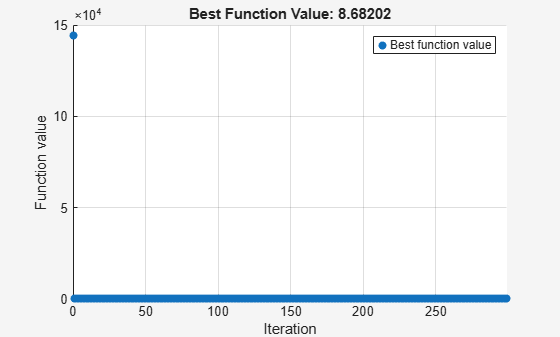```surrogateopt stopped because it exceeded the function evaluation limit set by 'options.MaxFunctionEvaluations'. ```

Set similar options for `patternsearch`, including a plot function to monitor the optimization.

```psopts = optimoptions('patternsearch','PlotFcn','psplotbestf','MaxFunctionEvaluations',mf); [psol,pfval] = patternsearch(fun,x0,[],[],[],[],lb,ub,[],psopts);```
```Maximum number of function evaluations exceeded: increase options.MaxFunctionEvaluations. ```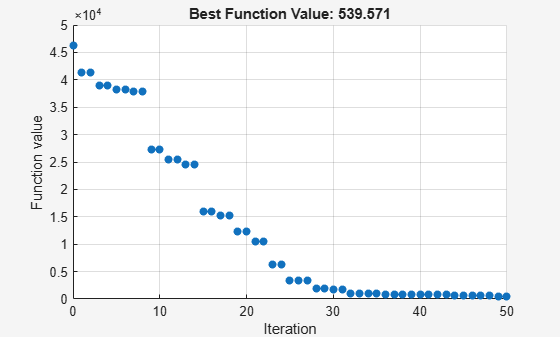Set similar options for `fmincon`.

```opts = optimoptions('fmincon','PlotFcn','optimplotfval','MaxFunctionEvaluations',mf); [fmsol,fmfval,eflag,fmoutput] = fmincon(fun,x0,[],[],[],[],lb,ub,[],opts);```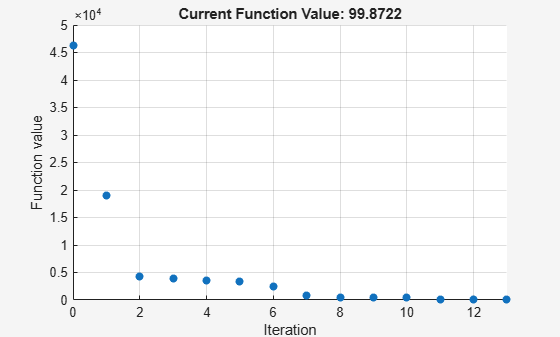```Solver stopped prematurely. fmincon stopped because it exceeded the function evaluation limit, options.MaxFunctionEvaluations = 2.000000e+02. ```

For this extremely restricted number of function evaluations, the `surrogateopt` solution is closest to the true minimum value of `0`.

`table(fvalm,pfval,fmfval,'VariableNames',{'surrogateopt','patternsearch','fmincon'})`
```ans=1×3 table surrogateopt patternsearch fmincon ____________ _____________ _______ 9.9646 860.28 493.7 ```

Allowing another 200 function evaluations shows that the other solvers rapidly approach the true solution, while `surrogateopt` does not improve significantly. Restart the solvers from their previous solutions, which adds 200 function evaluations to each optimization.

```options = optimoptions(options,'InitialPoints',pop); [xm,fvalm,~,~,pop] = surrogateopt(fun,lb,ub,options);```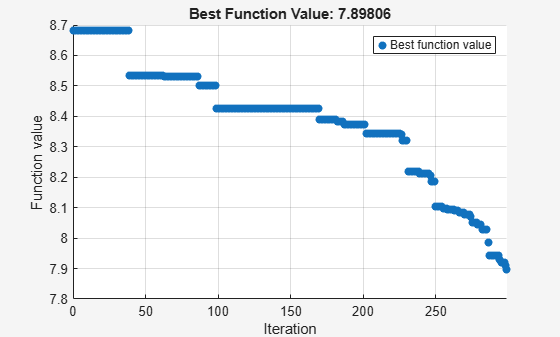```surrogateopt stopped because it exceeded the function evaluation limit set by 'options.MaxFunctionEvaluations'. ```
`[psol,pfval] = patternsearch(fun,psol,[],[],[],[],lb,ub,[],psopts);`
```Maximum number of function evaluations exceeded: increase options.MaxFunctionEvaluations. ```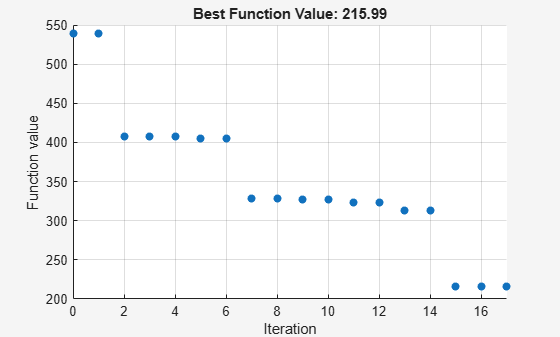`[fmsol,fmfval,eflag,fmoutput] = fmincon(fun,fmsol,[],[],[],[],lb,ub,[],opts);`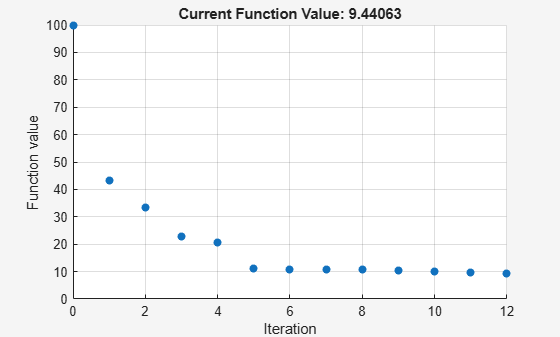```Solver stopped prematurely. fmincon stopped because it exceeded the function evaluation limit, options.MaxFunctionEvaluations = 2.000000e+02. ```
`table(fvalm,pfval,fmfval,'VariableNames',{'surrogateopt','patternsearch','fmincon'})`
```ans=1×3 table surrogateopt patternsearch fmincon ____________ _____________ _______ 9.7874 407.88 8.5989 ```